# Distance sampling simulations

Using dsims to investigate truncation distances with individual level covariates.

Laura Marshall http://distancesampling.org (CREEM, Univ of St Andrews)https://creem.st-andrews.ac.uk
2022-08-17

## Preamble

The first version of the simulation engine was a package called DSsim ; with improving GIS capabilities in R we have later released a second more efficient simulation package, dsims . This vignette was originally written for DSsim and so we use it now to not only demonstrate dsims but also as an example for users of DSsim showing how to transition to dsims. As the two packages have largely the same function names loading them together is not advised, this vignette will therefore leave the DSsim code in as comments for comparison with the dsims code. Please note that normal comments follow a single # and DSsim code follows double ##. It should also be noted that dsims now uses the distance sampling survey design package, dssd , to generate the transects based on the users specified design so that shapefiles containing the transects no longer need to be created in advance.

If your goal is to transition to dsims from DSsim then you will find all you need in the sections up to and including the section on running simulations. The latter sections go on to run a series of additional simulations investigating pooling robustness and covariate parameter estimation with respect to truncation distance. If you are completely new to distance sampling simulations then an alternative place to start is the Getting Started vignette inside the dsims package. This vignette uses dsims to compare a systematic parallel design with a zigzag design to assess the accuracy/precision trade off. To view this open R and after installing dsims, enter the following code:

vignette("GettingStarted", package = "dsims")

## Introduction

Distance sampling is a process in which a study area is surveyed to estimate the size of the population within it. It can be thought of as an extension to plot sampling. However, while plot sampling assumes that all objects within the plots are detected, distance sampling relaxes this assumption. To do this Distance sampling makes an assumptions about the distribution of objects with respect to the transects and to satisfy these assumptions the transects (the points or lines) must be randomly located within the study region. Note that for the purposes of distance sampling an object can either be an individual or a cluster or individuals.

The next step in distance sampling is then to record the distances from each detected object to the transect it was detected from and fit a detection function. From this function we can estimate how many objects were missed and hence the total number in the covered area. For example, Figure 1 shows histograms of distances that might be collected on a line transect survey, with a fitted detection function. If the lines have been placed at random within the study region then we would expect on average the same number of object to occur at any given distance from the transect. Therefore the drop in number of detection with increasing distance from the line can be attributed to a failure to detect all objects. We can therefore estimate from this detection function that the probability of seeing an object within the covered region out to a chosen truncation distance is the area under the curve (shaded grey) divided by the area of the rectangle.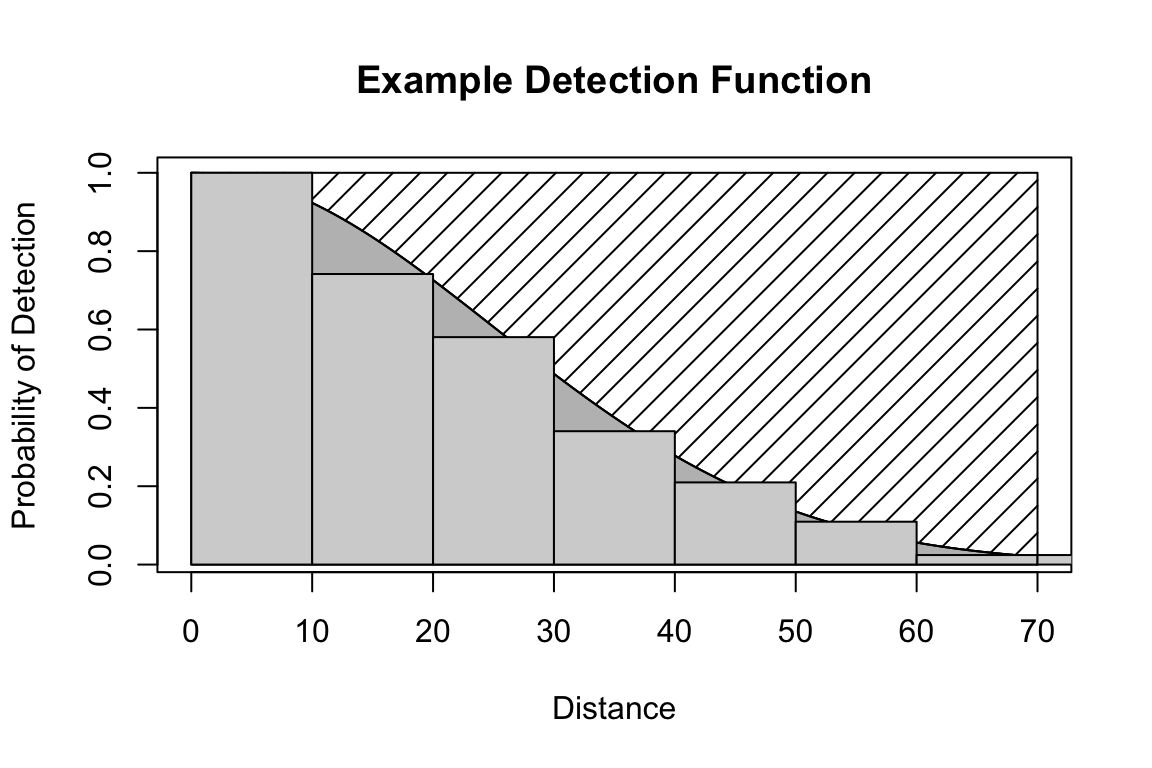Figure 1: An example detection function. The histogram shows example distances recorded from a line transect. The smooth curve is the detection function. The grey shaded area represents the number of detected objects and the diagonal hash region represents the number of objects in the covered region that were not detected.

The R package dsims allows users to simulate both point and line transect surveys, and test out a range of design and analysis decisions specific to their population of interest. To simulate surveys the user must make some assumptions about the population of interest and the detection process giving rise to the observed distances. Simulations can be repeated over a range of assumptions so that the user can be confident that their chosen design will perform well despite any uncertainty.

### Introduction to dsims

dsims takes information from the user on the study region, population and detection process and uses it to generate distance sampling data. dsims can then be asked to fit detection functions to this data and produce estimates of density, abundance and the associated uncertainty. dsims splits this process into three stages. Firstly, it generates an instance of a population and a set of survey transects. Secondly, it simulates the distance sampling survey using the assumed detection function(s) provided by the user. Lastly, dsims analyses the data from the survey. Figure 2 illustrates the simulation process and highlights the information which must be provided by the user.

Distance sampling simulations can be very useful to researchers who wish to optimise their survey design for their specific study regions and species of interest in order to try and achieve the most accurate / precise estimates for their populations. Setting up and running such simulations to optimise a design is a very small cost in comparison to those associated with actually completing the survey!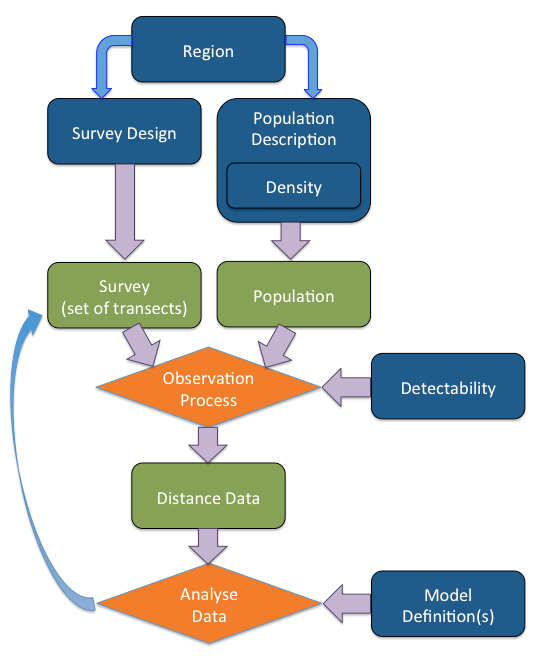Figure 2: Illustrates the simulation process. Blue rectangles indicate information supplied by the user. Green rectangles are objects created by dsims in the simulation process. Orange diamonds indicate the processes carried out by dsims.

dsims is written using the S4 object orientated system in R. The S4 system is a more formal and rigorous style of object orientated programming than the more commonly implemented S3. The process of defining a simulation involves the specification of many variables relating to the survey region, population, survey design and finally the analysis. The design of dsims is based around each of these descriptions being contained in its own class and the formal S4 class definition procedure ensures that the objects created are of the correct format for the simulation. As the objects created by dsims are instances of S4 classes, if the user wishes to access information within them the symbol used is slightly different. To access named parts of S3 objects the “$” symbol would be used, while for S4 objects the “@” symbol must be used. The following code demonstrates this. # load simulation package ## library(DSsim) library(dsims) # Make a default region object ## eg.region <- make.region() eg.region <- make.region() # Let's check the structure of the object we have created str(eg.region) Formal class 'Region' [package "dssd"] with 5 slots ..@ region.name: chr "region" ..@ strata.name: chr "region" ..@ units : chr(0) ..@ area : num 1e+06 ..@ region :Classes 'sf' and 'data.frame': 1 obs. of 2 variables: .. ..$ region  : chr "study_ar"
.. ..$geometry:sfc_POLYGON of length 1; first list element: List of 1 .. .. ..$ : num [1:5, 1:2] 0 0 2000 2000 0 0 500 500 0 0
.. .. ..- attr(*, "class")= chr [1:3] "XY" "POLYGON" "sfg"
.. ..- attr(*, "sf_column")= chr "geometry"
.. ..- attr(*, "agr")= Factor w/ 3 levels "constant","aggregate",..: NA
.. .. ..- attr(*, "names")= chr "region"
# If we wanted to extract the area of the region we would use
eg.region@area
 1e+06

### Example Simulation Study: Which Truncation Distance?

It is usual in distance sampling studies to truncate the data at some distance from the transect. This is because the observations far away from the transect are of lesser importance when fitting the detection function and also these sparse observations at large distances could have high influence on model selection and possibly increase variability in estimated abundance / density.

Buckland et al. (2001) suggest truncating the data where the probability of detection is around 0.15 as a general rule of thumb. However, distance sampling data is often costly to obtain and discarding some of the data points can feel counter intuitive. In this vignette we investigate truncation distance in distance sampling analyses. We will do this through a series of three simulations outlined below.

Firstly, this vignette will investigate data generated assuming a simple half normal detection function where every object has the same probability of detection at a specific distance from the transect. Figure 3 shows a simple half normal detection function with three possible truncation distances at $$1*\sigma$$, $$2*\sigma$$ and $$3*\sigma$$ where $$\sigma$$ is the scale parameter of the half normal detection function. The truncation distance at $$2*\sigma$$ gives a probability of detection of 0.135 so close to the 0.15 rule of thumb.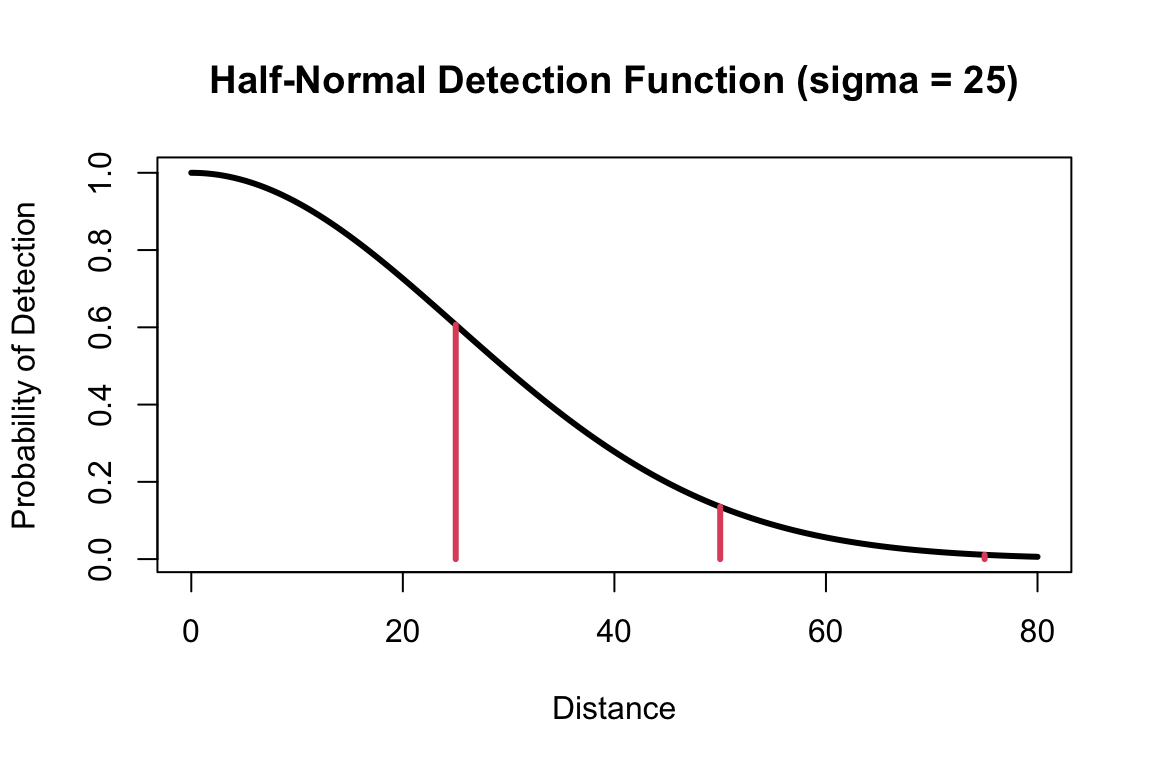Figure 3: Half-normal detection function showing 3 proposed truncation distances at $$1*\sigma$$, $$2*\sigma$$ and $$3*\sigma$$. The truncation distance at twice sigma gives a probability of detection of 0.135 so close to the 0.15 rule of thumb.

While the first set of simulations assume a simple half normal detection function, in reality individual objects or clusters of objects will likely have varying probability of being detected based on certain characteristics. Perhaps the behaviour of males will make them easier to detect. It is also easy to see that larger clusters of individuals might be easier to spot at large distances than small clusters. We will also investigate the effects of truncation distance when individual level covariates affect the probability of detection. Figure 4 shows how covariates may affect detectability. We will use simulated distance data with one covariate (sex) to investigate both the effects of truncation when we assume that we were not able to measure the covariate affecting detectability and when we assume that we can and therefore will include the relevant covariate in the detection function model.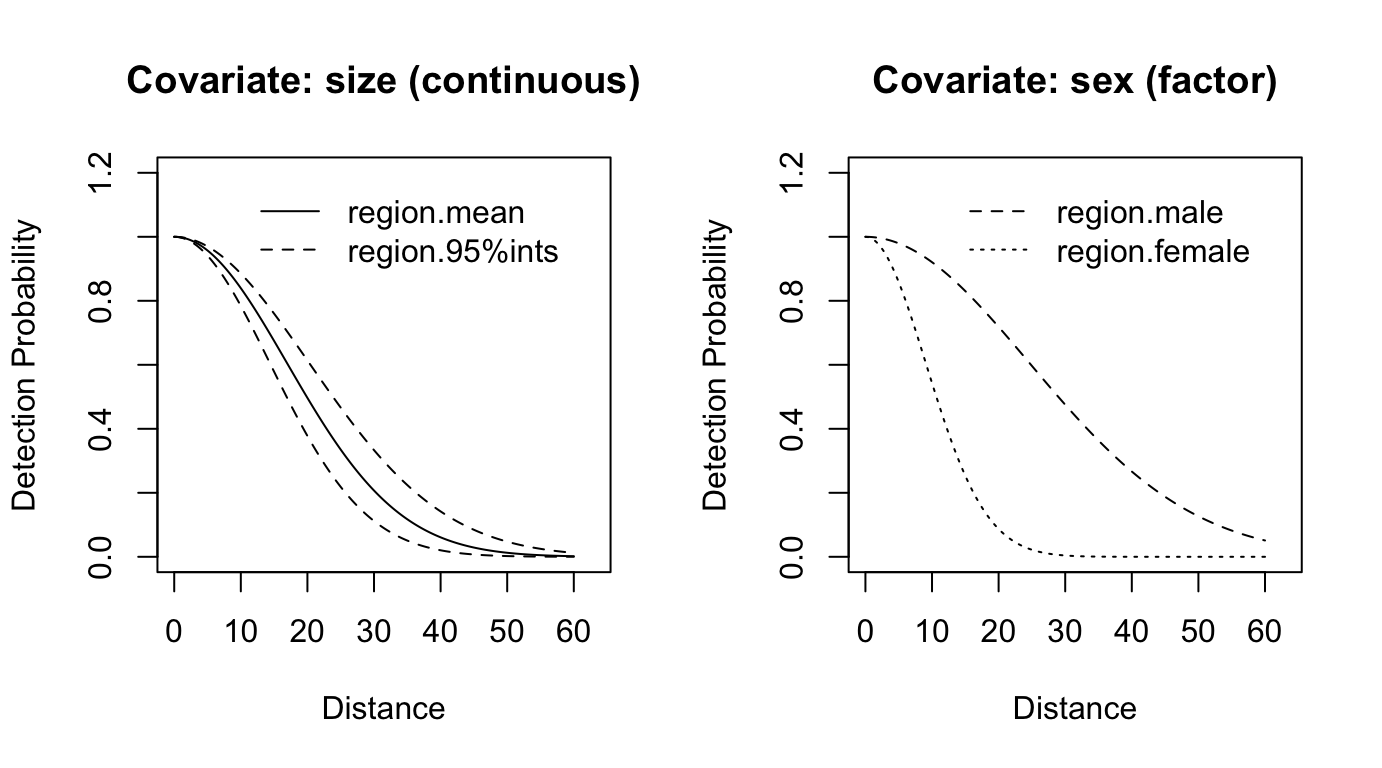Figure 4: Half-normal detection function which varies based on cluster size and animal sex.

### Model Uncertainty and Pooling Robustness

When we simulate data, we have to provide the detection function to generate detections, and we therefore know the underlying true detection function. When collecting data in the field, we will not have this information, and so we will have to rely on some form of model selection. One method of model selection is to compare information criterion, dsims allows the user to select either AIC, AICc or BIC as the model selection criteria. For these simulations we will use AIC and allow dsims to select between a half-normal and a hazard rate model in the first two sets of simulations.

In addition, if the probability of detection is affected by covariates then we may not only have a single underlying detection function but a combination of detection functions giving rise to our observed data. In this situation we can either model detectability as a function of these covariates or rely on a concept called pooling robustness. Pooling robustness refers to the fact that distance sampling techniques are robust to the pooling of multiple detection functions into one. This means that we do not necessarily need to include all the covariates which affect detectability in the detection function to accurately estimate density / abundance. This vignette will examine the concept of pooling robustness to see if it is affected by truncation distance.

## Methods

This vignette will guide you through the steps to create and run a series of simulations to investigate the effects of varying truncation distance on both data generated from a simple half-normal detection function and from a detection function where detectability is affected by a covariate.

## Setup

First we load the dsims library.

## library(DSsim)
library(dsims)

## Simulation Components

As detailed in Introduction to dsims a simulation comprises of a number of components. dsims is designed so that each of these components is defined individually before they are grouped together into a simulation. This helps keep the process clear and also allows reuse of simulation components between different simulations. Each of the function names to create a simulation component or simulation takes the form make.<component>.

### Region

These simulations will use a rectangular study region of 5 km by 20 km. Survey regions can be defined in any units but all units must be the same throughout the components of the simulation. If a shapefile is used to create the survey region, then information on the units will be taken from the .prj file. Here we will define the coordinates in m. As this is a simple study region (Figure 5) with few vertices we can simply provide the coordinates. A change from DSsim is that you now need to turn the coordinates into an sf polygon shape prior to creating the region. This step is documented below. Note that while standard shapefiles have their outer polygon coordinates given in a clockwise direction, sf uses counter clockwise for external polygons and clockwise for holes within polygons. Further details on creating multi-part or multi-strata sf objects can be found at the end of the multi-strata dssd vignette.

You will also note that units are no longer a plotting option. The plot functions have been modified to use ggplot2 and if additional plotting options are desired the ggplot object can be captured and modified.

## # Create a polgon
## poly1 <- data.frame(x = c(0,0,20000,20000,0), y = c(0,5000,5000,0,0))
##
## # Create an empty list
## # Store the polygon inside a list in the first element of the coords list referring to strata 1.
## coords <- list()
## coords[] <- list(poly1)

# Create an sf polgon
library(sf)
# Put the coordinates of the polygon in a matrix
poly1 = matrix(c(0,0, 20000,0, 20000,5000, 0,5000, 0,0),ncol=2, byrow=TRUE)
# Turn them into an sf polygon
pl1 = st_polygon(list(poly1))

## # Create the survey region
## region <- make.region(region.name = "study area",
##                       units = "m",
##                       coords = coords)
## # The plot function allows plotting in km or m.
## plot(region, plot.units = "km")

# Create the survey region
region <- make.region(region.name = "study area",
units = "m",
shape = pl1)
# The plot function allows plotting in km or m.
plot(region)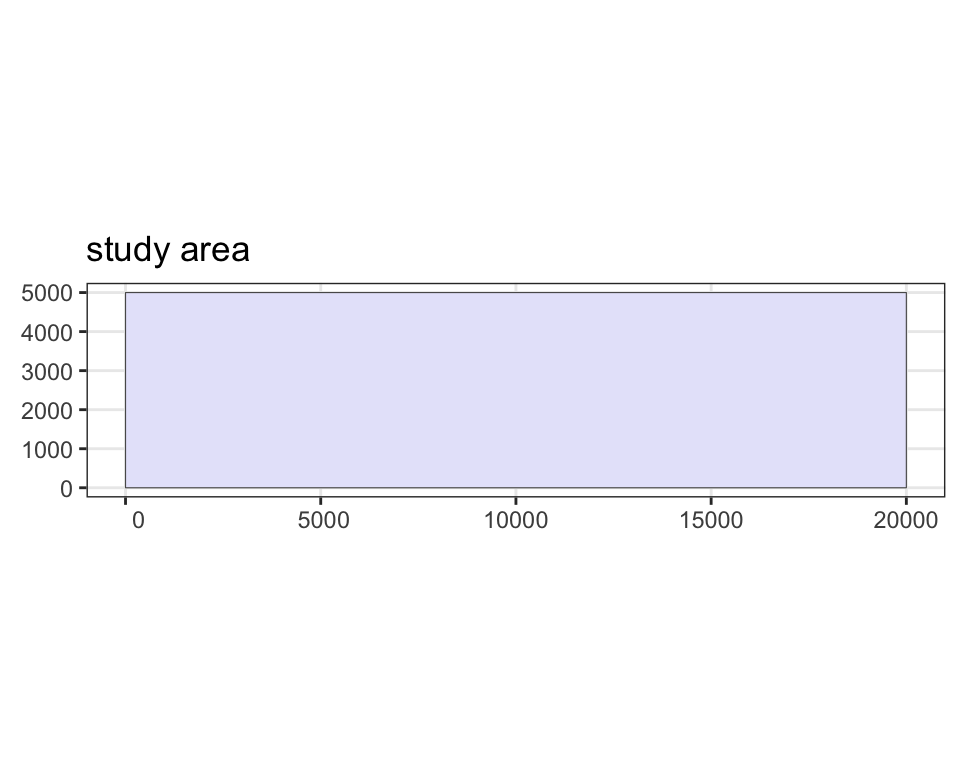Figure 5: The study region.

### Population

We will now define our population within our study region. Firstly, we must describe the distribution of the population by defining a density surface. For these simulations we will assume a uniform distribution of animals throughout the study region. dsims will generate an sf grid describing the density surface for us if we provide the x (and optionally the y) spacing and a constant density value for the surface. If the y spacing is omitted it will be assumed to be equal to the x spacing. In this example the value of the constant is not important as we will generate animals based on a fixed population size rather than using the exact values in the density grid.

There are two argument name changes in the make.density function: region.obj is now region and density.gam is now fitted model. The buffer argument is no longer needed and there is now an option to supply a formula for density based on x and y using density.formula.

## # Create the density surface
## density <- make.density(region.obj = region,
##                         x.space = 100,
##                         constant = 1)
##
## # Plot the density surface
## plot(density, style = "blocks")
## plot(region, add = TRUE)

density <- make.density(region = region,
x.space = 100,
constant = 1)

# Plot the density surface
plot(density, region)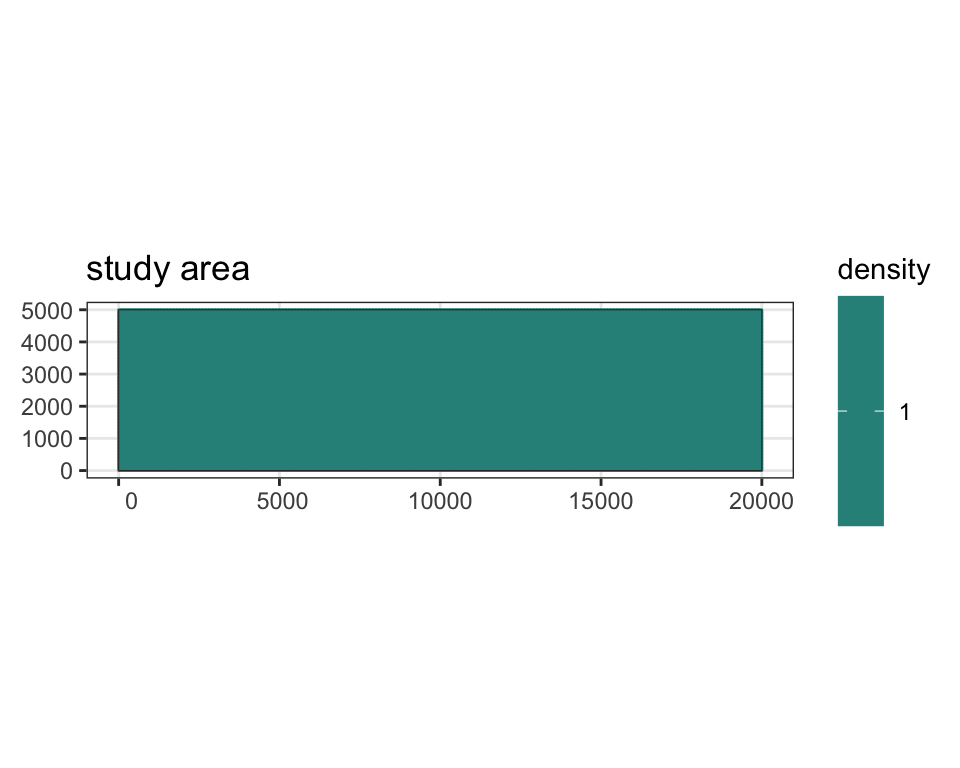Figure 6: The density surface.

As an aside, if we wished to add areas of higher or lower density to our density surface we could do this using the add.hotspot function in dsims. This function adds these hot or low spots based on a Gaussian decay function. We have to provide the central coordinates and a sigma value to tell dsims about the location and shape of the hot/low spot. The amplitude argument gives the value of the hot or low spot at its centre and is combined with the existing density surface through addition.

The code used to do this in dsims is identical to that used with DSsim and so is not repeated in the code chunk below.

# Add a hotspot to the density surface, centre located at x = 15000, y = 4000 with
# a Gaussian decay parameter sigma = 1500. The value at the centre point will now
# be 1 (the current value of the density surface defined above) + 0.5 = 1.5
eg.density <- add.hotspot(density, centre = c(15000,4000), sigma = 1500, amplitude = 0.5)
# Add a lowspot to this new density surface (eg.density)
eg.density <- add.hotspot(eg.density, centre = c(10000,3000), sigma = 1000, amplitude = -0.25)
# Plot the density surface
plot(eg.density, region)